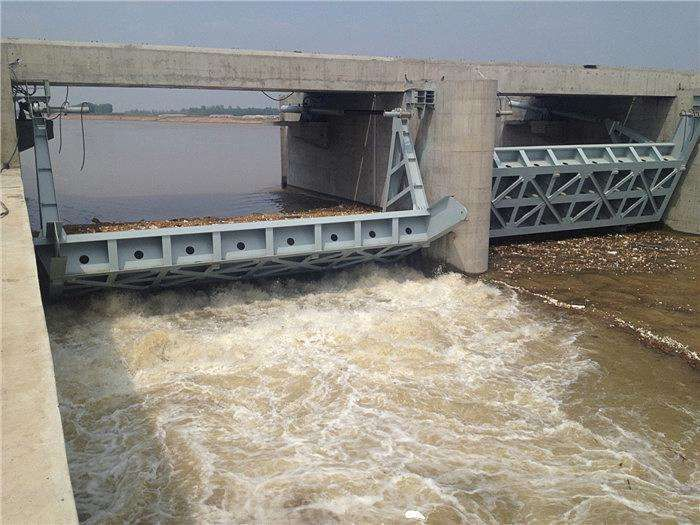深圳启闭机闸门厂家，本涵闸孔口尺寸 3×3m ，计 1 孔。采用平面钢闸门，门高 3.3m，Q235 实腹式多主梁焊接结构，焊条 E4303，悬臂式主滚轮支承。主滚轮材料 ZG310-570，主轴材料 45 号钢，轴衬材料 ZQAl9-4 铸铝青铜，侧向采用 MC油尼龙侧滑块，止水采用 P型及条形橡皮止水。采用QL-100KN 手电两用螺杆式启闭机启闭，计 1台。本工程闸门按远景行洪水位组合计算。水位组合：位置 项 目 上游 下游孔径计算 2.67 2.54稳定（设计） 2.20 7.42（近期）稳定（校核） 2.20 9.37（远景）消 能 2.67 1.67启闭门最大水位差按近期水位组合计算。2、面板计算闸门所受总水压力 P=733.4KN。面板计算厚度 δ=11.4mm,考虑面板腐蚀等因素，取δ=12mm。3、主梁计算主梁计算线载 q=118.5kN/m， L0=3.3m， L=3.1m， Mmax=160.8KN.m，Qmax=183.7KN。断面-10×372，-16×160，面板作用有效宽度B取 650mm。Wmin=1302773mm3Smax=1009111mm3应力 σmax=123.5N/mm2<[σ]=160N/mm2τmax=52.1N/mm2<[τ]=95N/mm2挠度 fmax=2.54mmfmax/L0=1/1299.2<[f/L]=1/750 钢闸门设计说明 24、主滚轮设计计算最大轮压 P=183.4kN（下滚轮） ，主轮半径 R=265mm，轮缘宽度b=100mm，轴套为 ZQAl9-4铸铝青铜。主轮计算:ZG310-570屈服点 σs =310N/mm2轨道 Q235钢屈服点σs =235N/mm2，取 Q235 钢 σs 值。σmax=0.418[1.1×P×E/(b×R)]0.5=523.5N/mm2<3.0σs =705N/mm2，满足要求。轮轴计算：材料45号钢，轮轴直径取d=150mm。q=2017.4kN/mMmax=26.23kN.m，Qmax=201.8kNσmax=79.21N/mm2<[σ]=145N/mm2τmax=15.3N/mm2<[τ]=95N/mm2,均满足要求。轴套计算：材料 ZQAl9-4铸铝铁青铜σcg=Pl/dbl=1.1×183400/(120×100)=16.82N/mm2<[σcg]=145N/mm2轴承板计算：选 14mm厚 Q235 钢板 2 块σcj=N/dΣδ=1.1×183400/(150×28)=48.1N/mm2<[σcj]=80N/mm2，满足要求。5、启闭力计算为确保工程安全，及增强闸门调控能力，按近期水位计算启闭力。远景水位时闸门处于关闭挡水状态。作用在闸门上总水压力 W=533.9kN作用在止水上总水压力 Pzs=17.23kN采用滑动轴承，滚轮 R=265mm，轮轴 r=60mm。滑动摩擦系数 f1=0.25（钢对铸铝青铜）滚动摩擦系数 fk=1mm。Tzd=30.22kN 钢闸门设计说明 3Tzs=12.06kN启门力 FQ=91.44KN，闭门力 Fw=17.44kN，持住力 Ft=-1.58kN。采用 QL-100KN螺杆启闭机启门，利用螺杆压力闭门。6、吊座计算吊轴 采用 Q235钢，[τ]=65N/mm2 采用单吊点。启吊力： P=100KN吊轴每边剪力： V=P/2=50KN需要吊轴截面积：A=V/[τ]=50×103/65=769mm2故吊轴直径 d ≥(A/0.785)1/2= 31.3mm取直径d=50mm吊耳板强度验算按局部紧接承压条件[σcj]=80N/mm2t=P/d[σcj]=100×103/50×80=25mm 取 t=28mm。吊耳孔壁拉应力：σk=σcj(R2+r2)/(R2-r2)式中 σcj=P/td=100×103/28×50=71.4N/mm2 <[σj]=80N/mm2σk=71.4(752+252)/(752-252)